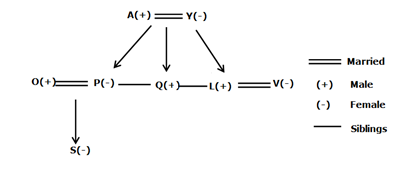# SBI Clerk Prelims Reasoning Ability Questions 2021 (Day-37)

Dear Aspirants, Our IBPS Guide team is providing new series of Reasoning Questions for SBI Clerk Prelims 2021 so the aspirants can practice it on a daily basis. These questions are framed by our skilled experts after understanding your needs thoroughly. Aspirants can practice these new series questions daily to familiarize with the exact exam pattern and make your preparation effective.

Start Quiz

Box based puzzle

Direction (1-5): Answer the questions based on the information given below.

Twelve boxes viz. A, B, C, D, E, F, G, H, I, J, K and L were placed in three different stacks viz. Stack 1, Stack 2 and Stack 3, but not necessarily in the same order. Stack 1 is to the west of Stack 2 and Stack 3 is to the east of Stack 2. The lowermost box is numbered 1 and the box immediately above is numbered 2 and so on.

G was placed in an even numbered box of Stack 2 and immediately above J. F was placed to the east of J and two boxes away from I on the same stack. E was placed three boxes above D but on the adjacent stack to the east of D.L was placed two boxes below H on the same stack and H was placed on the same box as E. A was placed to the extreme east of L.B was placed to the west of K where C was placed to the northeast of K.

1) Which of the following box was placed immediately above L on the same stack?

A.K

B.D

C.B

D.C

E.None of these

2) How many boxes are there between A and F?

A.None

B.Three

C.Five

D.Two

E.None of these

3) In which of the following position does K was placed?

A.On the same box as A

B.On the same stack as L

C.Immediately below E

D.On the bottommost position

E.None of these

4) Which of the following statement is true with respect to the final arrangement?

A.I was placed in stack 2

B.Both D and E are placed in the odd positions

C.K was placed in the topmost position

D.J was placed two boxes below B on different stacks

E.None of these

5) Four of the following five are alike in a certain way and hence form a group. Find the one that doesn’t belong to that group.

A.K

B.G

C.J

D.E

E.F

Direction sense

Direction (6-8): Study the following information carefully and answer the below questions

Point T is north of point X and east of point H. Point X is north of Point M. Point S is south of Point V. Point P is west of Point S and south of Point H. Point V is east of point M.

6) If TM=10km, VS=6km, then the distance between the points H and P?

A.15km

B.12km

C.16km

D.4km

E.None of these

7) What is the direction of point P with respect to point T?

A.East

B.North

C.North-West

D.South-east

E.South-west

8) Four of the following are alike in a certain way. Which of thefollowing does not belong to the group?

A.VS

B.TX

C.HP

D.TM

E.VP

Blood relation

Direction (9-10): Study the following information carefully and answer the below questions.

A family consisting of eight members and three married couples. P is the only daughter of A, who is the father of L. Y has three children. Q is the bother-in-law of O who is the father of S. S is the niece of L. V is the daughter-in-law of Y. Q is unmarried.

9) How L is related to A?

A.Son

B.Daughter

C.Nephew

D.Niece

E.Can’t be determined

10) How many daughters are there in the family?

A.0

B.3

C.2

D.1

E.Can’t be determined.

Directions (1-5):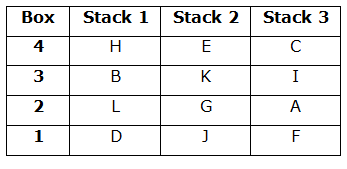1) G was placed in an even numbered box of Stack 2 and immediately above J. F was placed to the east of J and two boxes away from I on the same stack.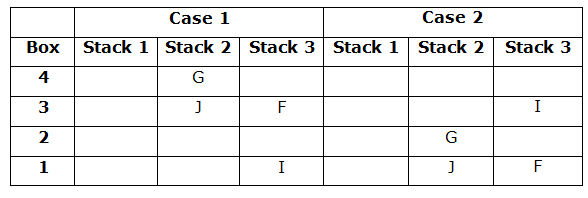2) E was placed three boxes above D but on the adjacent stack to the east of D. L was placed two boxes below H on the same stack and H was placed on the same box as E.A was placed to the extreme east of L.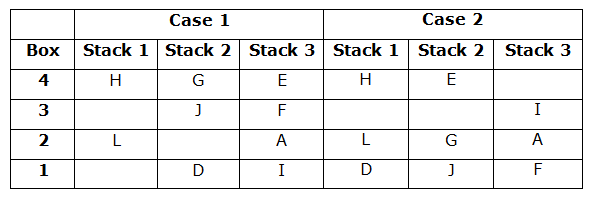3) B was placed to the west of K where C was placed to the northeast of K. Hence, case 1 gets eliminated.Directions (6-8) :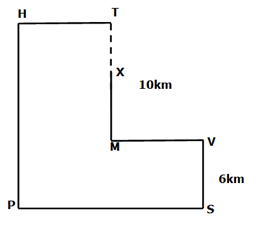Directions (9-10) :